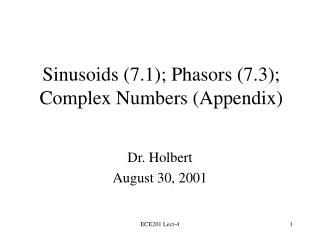Download PresentationSinusoids (7.1); Phasors (7.3); Complex Numbers (Appendix)

# Sinusoids (7.1); Phasors (7.3); Complex Numbers (Appendix) - PowerPoint PPT PresentationDownload Presentation## Sinusoids (7.1); Phasors (7.3); Complex Numbers (Appendix)

- - - - - - - - - - - - - - - - - - - - - - - - - - - E N D - - - - - - - - - - - - - - - - - - - - - - - - - - -
##### Presentation Transcript

1. Sinusoids (7.1); Phasors (7.3);Complex Numbers (Appendix) Dr. Holbert August 30, 2001 ECE201 Lect-4

2. Introduction • Any steady-state voltage or current in a linear circuit with a sinusoidal source is a sinusoid. • This is a consequence of the nature of particular solutions for sinusoidal forcing functions. • All steady-state voltages and currents have the same frequency as the source. ECE201 Lect-4

3. Introduction (cont.) • In order to find a steady-state voltage or current, all we need to know is its magnitude and its phase relative to the source (we already know its frequency). • Usually, an AC steady-state voltage or current is given by the particular solution to a differential equation. ECE201 Lect-4

4. The Good News! • We do not have to find this differential equation from the circuit, nor do we have to solve it. • Instead, we use the concepts of phasorsand complex impedances. • Phasors and complex impedances convert problems involving differential equations into simple circuit analysis problems. ECE201 Lect-4

5. Phasors • A phasor is a complex number that represents the magnitude and phase of a sinusoidal voltage or current. • Remember, for AC steady-state analysis, this is all we need---we already know the frequency of any voltage or current. ECE201 Lect-4

6. Complex Impedance • Complex impedance describes the relationship between the voltage across an element (expressed as a phasor) and the current through the element (expressed as a phasor). • Impedance is a complex number. • Impedance depends on frequency. ECE201 Lect-4

7. Complex Impedance (cont.) • Phasors and complex impedance allow us to use Ohm’s law with complex numbers to compute current from voltage and voltage from current. ECE201 Lect-4

8. Sinusoids • Period: T • Time necessary to go through one cycle • Frequency: f = 1/T • Cycles per second (Hz) • Radian frequency: w = 2pf • Amplitude: VM ECE201 Lect-4

9. Example What is the amplitude, period, frequency, and angular (radian) frequency of this sinusoid? ECE201 Lect-4

10. Phase ECE201 Lect-4

11. Leading and Lagging Phase x1(t) leads x2(t) by q- x2(t) lags x1(t) by q- On preceding plot, which signals lead and which lag? ECE201 Lect-4

12. Class Examples • Learning Extension E7.1 • Learning Extension E7.2 ECE201 Lect-4

13. Phasors • A phasor is a complex number that represents the magnitude and phase of a sinusoidal voltage or current: ECE201 Lect-4

14. Phasors (cont.) • Time Domain: • Frequency Domain: ECE201 Lect-4

15. Summary of Phasors • Phasor (frequency domain) is a complex number: X = zq = x + jy • Sinusoid is a time function: x(t) = z cos(wt + q) ECE201 Lect-4

16. Class Examples • Learning Extension E7.3 • Learning Extension E7.4 ECE201 Lect-4

17. Complex Numbers • x is the real part • y is the imaginary part • z is the magnitude • q is the phase imaginary axis y z q real axis x ECE201 Lect-4

18. More Complex Numbers • Polar Coordinates: A = z q • Rectangular Coordinates: A = x + jy ECE201 Lect-4

19. Are You a Technology “Have”? • There is a good chance that your calculator will convert from rectangular to polar, and from polar to rectangular. • Convert to polar: 3 + j4 and -3 - j4 • Convert to rectangular: 2 45 & -2 45 ECE201 Lect-4

20. Arithmetic With Complex Numbers • To compute phasor voltages and currents, we need to be able to perform computation with complex numbers. • Addition • Subtraction • Multiplication • Division ECE201 Lect-4

21. Complex Number Addition and Subtraction • Addition is most easily performed in rectangular coordinates: A = x + jy B = z + jw A + B = (x + z) + j(y + w) • Subtraction is also most easily performed in rectangular coordinates: A - B = (x - z) + j(y - w) ECE201 Lect-4

22. Complex Number Multiplication and Division • Multiplication is most easily performed in polar coordinates: A = AMq B = BMf A B = (AM  BM)  (q + f) • Division is also most easily performed in polar coordinates: A / B = (AM / BM)  (q - f) ECE201 Lect-4

23. Examples • Find the time domain representations of V = 104V - j60V I = -1mA - j3mA at 60 Hz • If Z = -1 + j2 , then find the value of IZ + V ECE201 Lect-4